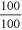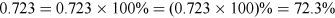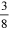Succeed with maths – Part 1

Start this free course now. Just create an account and sign in. Enrol and complete the course for a free statement of participation or digital badge if available.

1.2 Writing a decimal as a percentage

Because you divide a percentage by 100 to convert it into a decimal, you may assume that in order to change a decimal to a percentage, you must multiply by 100 (because multiplication undoes division). And you would be correct to assume this! A quick way to do this is to move the decimal point two places to the right.

To show that this works, use a calculator to work out 0.005 x 100. The answer should be 0.5 (that is, 0.5 per cent), the same as moving the decimal point two places to the right, as expected.

If you want to convert 1.245 into a percentage, you need to multiply by 100. So, 1.245 = 124.5 per cent.

Let’s take a closer look at this to make sure that this makes mathematical sense. ‘Per cent’ means ‘over 100’, so an equivalent way of expressing ‘100 per cent’ is, which is the number 1. Multiplying by 100 per cent does not change the value of a number; it just makes it look different.

If you like, you can show the conversion with one or both of the following steps:.

Now you need to look at writing fractions as a percentage. This can be useful, because many people will have a much better understanding of what a percentage means rather than a fraction. For example, you could say that, or 37.5 per cent, of the population prefers vanilla ice cream. For most people, the percentage would mean more.